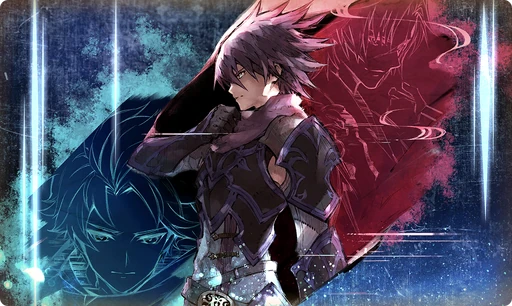# Where Our Heart Leads Us

Type
Equipment
Rank
5★
Max level
40
Enhancer cost
2000
Enhancer EXP
20000
Source
Unreleased
AlchemistCodeDBFor the Wadatsumi he loved. For the world he must protect. His oath and his bonds were his mission, and so much more. I always wondered, "What does it mean to be strong?" I had nothing in this world. I chose to grasp the hand that stretched out to me. I'm sure I wasn't the only one to do so. (Basheeny's Recollections)

## Stats

#### Simple stats

TypeLvl 1Lvl 30Lvl 40
HP+30+30+30

LevelHP
1+30
2+30
3+30
4+30
5+30
6+30
7+30
8+30
9+30
10+30
LevelHP
11+30
12+30
13+30
14+30
15+30
16+30
17+30
18+30
19+30
20+30
LevelHP
21+30
22+30
23+30
24+30
25+30
26+30
27+30
28+30
29+30
30+30
LevelHP
31+30
32+30
33+30
34+30
35+30
36+30
37+30
38+30
39+30
40+30

## Group skills

### Crowd Spirit

#### Simple stats

TypeLvl 1Lvl 30Lvl 40
PATK+10+10+10
PDEF+10+10+10
MATK+10+10+10
MDEF+10+10+10

#### Detailed stats

LevelPATKPDEFMATKMDEF
1+10+10+10+10
2+10+10+10+10
3+10+10+10+10
4+10+10+10+10
5+10+10+10+10
6+10+10+10+10
7+10+10+10+10
8+10+10+10+10
9+10+10+10+10
10+10+10+10+10
LevelPATKPDEFMATKMDEF
11+10+10+10+10
12+10+10+10+10
13+10+10+10+10
14+10+10+10+10
15+10+10+10+10
16+10+10+10+10
17+10+10+10+10
18+10+10+10+10
19+10+10+10+10
20+10+10+10+10
LevelPATKPDEFMATKMDEF
21+10+10+10+10
22+10+10+10+10
23+10+10+10+10
24+10+10+10+10
25+10+10+10+10
26+10+10+10+10
27+10+10+10+10
28+10+10+10+10
29+10+10+10+10
30+10+10+10+10
LevelPATKPDEFMATKMDEF
31+10+10+10+10
32+10+10+10+10
33+10+10+10+10
34+10+10+10+10
35+10+10+10+10
36+10+10+10+10
37+10+10+10+10
38+10+10+10+10
39+10+10+10+10
40+10+10+10+10